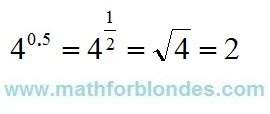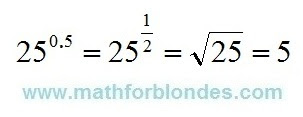## 10/30/2021

### 2 to the power zero five

How much is 2 to the power zero five? A trick question, like everything mathematicians come up with for us. In short, transformations look like this.2 to the power zero five

Now let's take a closer look at the logic of mathematicians. Raising a number to an integer power is the multiplication of these numbers. Raising a number to a fractional power is extracting the root of a number, with the number in the denominator showing which root to find. But the fraction itself can be written both in the form of a decimal fraction, and in the form of a regular fraction. We put all this in a pot, mix thoroughly and the mathematical puzzle for the textbook is ready.

You need to solve this puzzle in the following order. We convert the decimal fraction zero five into a regular fraction and get one second. Now we can write the number 2 with a fractional exponent as the square root of two and calculate its value.

To consolidate knowledge, consider two more simple examples.4 to the power zero five

4 to the power zero five is the same as 4 to the one-second power. Four to the power of one second is the square root of 4. Taking the square root of four gives two.25 to the power zero five

After considering the two previous examples, it will be very easy to calculate 25 to the power zero five. As real shamans, we turn zero five into one second, extract the square root of 25 and get the desired result - the root of twenty-five is five.

Why all these dances of shamans with tambourines? There are two sides to this math medal. Front side - mathematicians teach us to use mathematics. The other side of the coin is that if mathematicians simply and clearly express their thoughts in the language of mathematics, then they will not seem so smart to us, and we ourselves will not look such fools.

## 10/16/2021

How to find 101 cubed using abbreviated multiplication formulas? I have already shown how, using the cubes of the sum formula, one can calculate one hundred and one in a cube. There I presented one number 101 as the sum of two numbers 100 and 1. The use of simpler (more convenient in calculations) numbers makes the calculation easier.

On the Internet, I saw an example of using other formulas, the sum of cubes and the difference of cubes. This is a bad example. To find 101 cubed, use the numbers 101 and 100. This is how it looks.101 cubed. Bad example.

Why is this a bad example? I'll show you now. I'll take the number 101, the cube of which we need to find, and zero.101 cubed. Removing garbage.

By using zero, I removed all the mathematical garbage from the calculations - these are four multiplications and four additions or subtractions.

## 7/25/2021

### Cube of 101

Today we will look at an example of how you can find one hundred and one cubed using the abbreviated multiplication formulas. In other words, how to raise cube of 101 using the abbreviated multiplication formula.

The following cry for help was heard in the comments:

Help please, problem (example) from the physics and mathematics school (Russia), grade 8:

101^3

I don't understand how to solve this with abbreviated multiplication formulas.

It is very easy to cube the number 101 - you need to type the number 101 on a calculator and multiply twice by the same number 101.

101^3=101*101*101=1030301

If you don't have a calculator at hand (you never know, the phone has just been stolen), then you can calculate on a piece of paper in a column (the picture will be at the end, like checking both the calculator and the formula).

## Cube of 101 We apply the cube of sum formula.

The problem statement says that you need to find the cube of 101 using the abbreviated multiplication formula. According to math teachers, everyone should know these formulas. Naive. Where to find this formula?

You can search the Internet for a cube of sum. Google to help you. You can find these formulas in a reference book on mathematics, in a textbook on mathematics, you can ask a classmate who knows the formulas for abbreviated multiplication by heart. The formula for the abbreviated multiplication we need is called cube of sum. You will see it below.

And now the answer to the most tricky question: how to get the sum of numbers from one number? It is necessary to expand this number into terms. From the point of view of mathematics, the number of terms can be any, but ... I found the abbreviated multiplication formulas for raising a sum to a cube only for two and three terms. The formula for the cube of the sum of three terms is very complicated, I wish you never to face such a thing. But the cube of the sum of the two terms looks nice. The basic principle of expansion into terms for applying abbreviated multiplication formulas is that numbers can be easily multiplied in your head without using a calculator. For the number 101, the best option would be 101 = 100 + 1. 100 and 1 numbers is easy to multiply without a calculator. Let's see what we get.Cube of 101

I don't know about you, but I couldn't do without a piece of paper. Yes, I wrote everything down in a line, not a column, but nevertheless. And in conclusion, let's check our solution by multiplying in a column on a piece of paper.Cube of 101 in a column on a piece of paper

Well, something like this. Why do you need all this? So that you know that you can multiply not only on a calculator or in a column, but sometimes you can effectively use abbreviated multiplication formulas. If, of course, you know them.